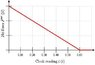# Net Impulse Question: Calculate Momentum, Impulse, and Change in Momentum

• hhq5000

## Homework Statement

The net force acting on a glider on an air track (an essentially frictionless system) varies with time as shown in the following diagram. The vertical scale is such that the space between consecutive vertical gridlines is 5.0 Newton(s).

The glider has a mass of 0.850 kg. When the force is applied to the glider at clock reading t = 0.00, the glider has an initial instantaneous velocity of 0.100 m/s in the positive direction of the x-axis.
(a) Calculate the momentum of the glider at t = 0.00 s (i.e., the initial momentum).
kg·m/s
(b) Calculate the net impulse imparted to the glider by the applied net force between t = 0.00 and t = 0.60 s.

N·s
(c) Calculate the change in momentum of the glider over this time interval.

(e) Calculate the instantaneous velocity of the glider at clock reading t = 0.60 s.
m/s

## Homework Equations

change in momentum= pf-pi

I=(Fav)(DeltaT)

## The Attempt at a Solution

i tried to use the the Impulse equation and did (15N)(.6seconds). I also attempted to use the change in momentum equation and was missing the velocity of the final. I need help with b-e.

#### Attachments

•43555.jpg
9 KB · Views: 595
first, the impulse is as you describe, but since the area is a triangle the total impulse is 1/2(15*.6)= 4.5N-s

I assume you had no problem computing the initial P. The change then is equal to the impulse, just as you have stated. Throw some numbers out and we'll see how that look.